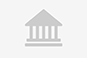# Learning Path: R: Master R Data Analysis and VisualizationTraining

Online

Descripción

• Tipología

Training

• Metodología

Online

• Horas lectivas

12h

• Inicio

Fechas disponibles

"R is one of the most comprehensible statistical tool for managing and manipulating data. With the ever increasing number of data, there is a very high demand of professionals who have got skills to analyze these data. If you're looking forward to becoming an expert data analyst, then go for this Learning Path.
Packt’s Video Learning Paths are a series of individual video products put together in a logical and stepwise manner such that each video builds on the skills learned in the video before it.
Let’s take a quick look at your learning journey! This Learning Path begins with familiarizing you with the programming and statistics aspects of R. You will learn how CRAN works and why to use it. Acquire the ability to conduct data analysis in practical contexts with R, using core language packages and tools.
Moving ahead, the Learning Path will gradually take you through creating interactive maps using the googleVis package. By the end of this Learning Path, you will be equipped with all data analysis and visualization techniques and build a strong foundation for moving into data science
We have combined the best authors:
Dr. Samik Sen is a theoretical physicist and loves thinking about hard problems. After his PH.D. in developing computational methods to solve problems for which no solutions existed, he began thinking about how to tackle math problems while lecturing.

Información importante

¿Qué objetivos tiene esta formación?: "Import and export data in various formats in R
Create simple and quick visualizations using the basic graphic tools in R
Implement interactive visualizations using ggplot2.
Add elements, text, animation, and colors to your plot to make sense of data
Master network, radial, and coxcomb plots"

¿Esta formación es para mi?: This Learning Path is aimed at aspiring or professional statisticians, data analysts, or data scientists who want to analyze and visualize data for gaining deeper insights of it.

Requisitos: "Basic programming knowledge of RBasic knowledge of Math and Statistics"

Sedes y fechas disponibles

Ubicación

comienzo

Online

comienzo

Fechas disponibles Inscripciones abiertas

Opiniones

Materias

• Data analysis
• Programming
• spatial
• Spatial Distribution
• Spatial points
• Spatial analysis
• Spatial Data
• R
• R Data
• Datanalysis
• Data Visualization
• R Studio
• Data
• Raster data
• Raster
• Raster format
• Vector
• Vector data
• Multivariate data
• Plotting

### Temario

"Speaking ‘R’ - The Language of Data Science
The Course Overview
What Is R?
Getting and Setting Up R/Rstudio
Using RStudio
Packages
A Lot Is the Same
Familiar Building Programming Blocks
Putting It All Together
Core R Types
Some Useful Operations
More Useful Operations
Titanic
Tennis
It's Mostly Cleaning Up
The Most Widely Used Statistical Package
Distributions
Time to Get Graphical
Plotting to Another Dimension
Facets
Learning Data Analysis with R
The Course Overview
Fixed-Width Format
Importing with read.lines (The Last Resort)
Importing Vector Data (ESRI shp and GeoJSON)
Transforming from data.frame to SpatialPointsDataFrame
Understanding Projections
Basic time/dates formats
Introducing the Raster Format
Mosaicking
Stacking to Include the Temporal Component
Exporting Data in Tables
Exporting Vector Data (ESRI shp File)
Exporting Rasters in Various Formats (GeoTIFF, ASCII Grids)
Exporting Data for WebGIS Systems (GeoJSON, KML)
Preparing the Dataset
Measuring Spread (Standard Deviation and Standard Distance)
Plotting for Multivariate Data
Finding Outliers
Introduction
Intersection
Buffer and Distance
Union and Overlay
Introduction
Converting Vector/Table Data into Raster
Subsetting and Selection
Filtering
Raster Calculator
Plotting Basics
Color Scale
Creating Multivariate Plots
Handling the Temporal Component
Introduction
Plotting Vector Data on Google Maps
Plotting Raster Data on Google Maps
Using Leaflet to Plot on Open Street Maps
Introduction
Importing Data from the World Bank
Concluding Remarks
Theoretical Background
Introduction
Intensity and Density
Spatial Distribution
Modelling
Theoretical Background
Data Preparation
K-Means Clustering
Optimal Number of Clusters
Hierarchical Clustering
Concluding
Theoretical Background
Subsetting and Temporal Functions
Decomposition and Correlation
Forecasting
Theoretical Background
Data Preparation
Mapping with Deterministic Estimators
Analyzing Trend and Checking Normality
Variogram Analysis
Mapping with kriging
Theoretical Background
Dataset
Linear Regression
Regression Trees
Support Vector Machines